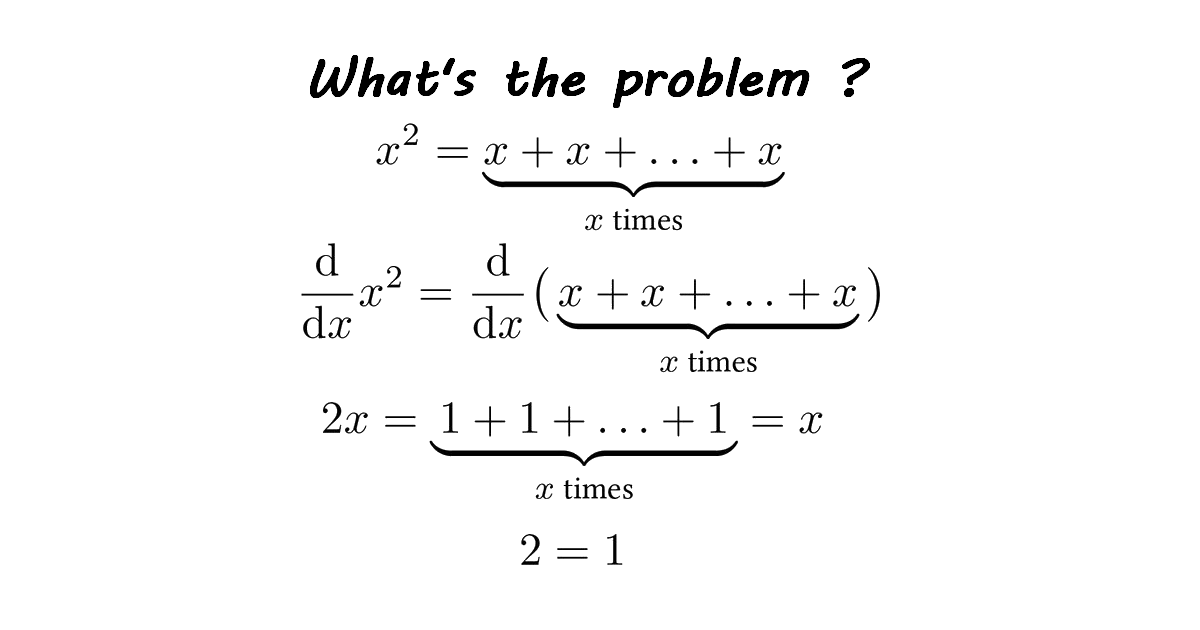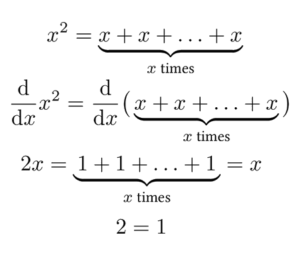Home -> Solved problems -> Derivative of x+x+…+x

## Derivative of x squared and derivative of x+x+...+x, x times### Solution

We can differentiate a function only if the function is continuous, when we write x²=x+x+…+x, x times it is not a continuous function. Thus, x+x+…+x, x times should not be differentiated.
Home -> Solved problems -> Derivative of x+x+…+x

### Related Topics

Find the volume of the square pyramid as a function of $$a$$ and $$H$$ by slicing method.
Prove that $\lim_{x \rightarrow 0}\frac{\sin x}{x}=1$
Calculate the half derivative of $$x$$
Prove Wallis Product Using Integration
Calculate the volume of Torus using cylindrical shells
Find the derivative of exponential $$x$$ from first principles
Calculate the sum of areas of the three squares
Find the equation of the curve formed by a cable suspended between two points at the same height
Solve the equation for real values of $$x$$
Solve the equation for $$x\epsilon\mathbb{R}$$
Calculate the following limit
Calculate the following limit
Prove that $$e$$ is an irrational number
Find the derivative of $$y$$ with respect to $$x$$
Find the limit of width and height ratio
Solve the equation for $$x \in \mathbb{R}$$
Is $$\pi$$ an irrational number ?
How far apart are the poles ?
Solve for $$x \in \mathbb{R}$$
What values of $$x$$ satisfy this inequality
Prove that the function $$f(x)=\frac{x^{3}+2 x^{2}+3 x+4}{x}$$ has a curvilinear asymptote $$y=x^{2}+2 x+3$$
Why does the number $$98$$ disappear when writing the decimal expansion of $$\frac{1}{9801}$$ ?
Only one in 1000 can solve this math problem
Error to avoid that leads to:
Home -> Solved problems -> Derivative of x+x+…+x

#### Share the solution: Derivative of x+x+...+x